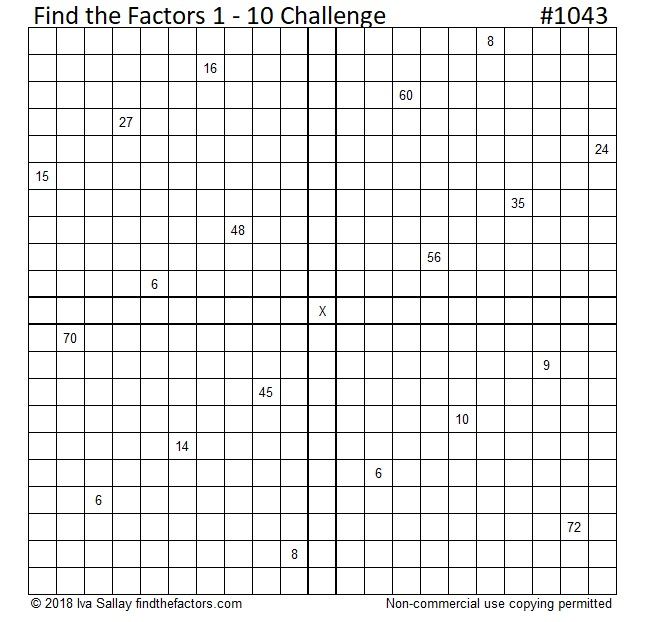# 1043 Find the Factors Challenge Puzzle

I made this particular Find the Factors 1-10 Challenge puzzle three weeks ago. It took me just under 30 minutes to solve it when I tried it again before publishing it. How long will it take you to solve it?Print the puzzles or type the solution in this excel file: 10-factors-1035-1043

Now here’s a little about the number 1043:

• 1043 is a composite number.
• Prime factorization: 1043 = 7 × 149
• The exponents in the prime factorization are 1 and 1. Adding one to each and multiplying we get (1 + 1)(1 + 1) = 2 × 2 = 4. Therefore 1043 has exactly 4 factors.
• Factors of 1043: 1, 7, 149, 1043
• Factor pairs: 1043 = 1 × 1043 or 7 × 149
• 1043 has no square factors that allow its square root to be simplified. √1043 ≈ 32.295511043 is the sum of consecutive prime numbers two different ways:
It’s the sum of the 21 prime numbers from 11 to 97 and,
it’s the sum of the 13 prime numbers from 53 to 107.

1043 is also the hypotenuse of a Pythagorean triple:
357-980-1043 which is 7 times (51-140-149)

This site uses Akismet to reduce spam. Learn how your comment data is processed.